Time

Write time in minutes rounded to one decimal place: 5 h 28 m 26 s.

Result

5 h 28 m 26 s =  328.4 min

Solution:Leave us a comment of this math problem and its solution (i.e. if it is still somewhat unclear...):Be the first to comment!Next similar math problems:

1. DayWhat part of the day is 23 hours 22 minutes? Express as a decimal number.
2. Videotape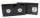Viera bought a videotape on which you can record programs with a total length of 240 minutes. She recorded a sci fi movie 1 hour and 28 minutes long, five ten-minute sessions "aerobics at home." Can she fits on the tape even film of Robin Hood who takes a
3. Fan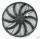The fan has a speed of 210 RPM. Calculate for time of one fan period.
4. Fruits 2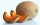An apple costs 7p and a melon costs seven times more. How much will 6 apples and 2 melons cost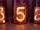What will be hexadecimal number 303 as decimal number?
6. Jumps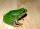At least how many jumps must do frog to overcome the distance of 7 meters? Jump of frog is 18 cm long.
7. Round it0.728 round to units, tenths, hundredths.
8. Crates 2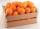One crate will hold 50 oranges. If Bob needs to ship 932 oranges, how many crates will he need?
9. RoundingThe following numbers round to the thousandth:
10. Combine / add termCombine like terms 4c+c-7c
11. EvaluateThe division of numbers 18 and 6 increase by product of the numbers 156 and 0.001
12. Double 5Peter was thinking of a number. Peter doubles it and gets an answer of 8.6. What was the original number?
13. Summerjobs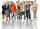The agency give summerjobs for 2352 students in 2018. The eighth was high-school students, the rest was undergraduates. How many undergraduates works via agency in 2018?
14. Division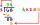Divide by the number 0.2 is the same as multiply by what number?
15. Evaluate expressionEvaluate expression using BODMAS rule: 1 1/4+1 1/5÷3/5-5/8
16. Farmer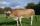The farmer farming 293 ha of arable land and the remaining 20% are meadows and pastures. How much land farmer owns?
17. Ratio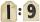Unfold number 1963 in the ratio 9:2:2.